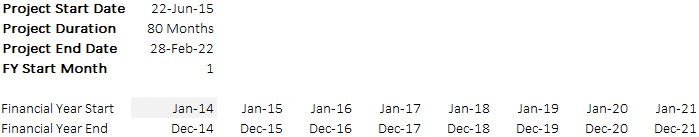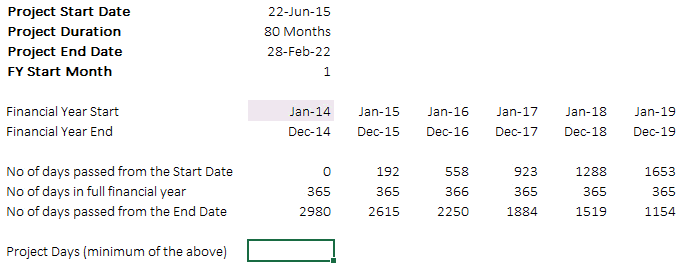Hi Guys, the second and the final post in the Time Scale series got a bit delayed, my sincere apologies. Let’s begin

#### Recap of our earlier post

In the earlier post we had seen how can we map the project period in a calendar and link that to our financial model. Do a quick review of the earlier post here – Time Scales in Financial Modeling Part 1

#### The Problem

Sometimes mere mapping of the relevant project period is not enough but we also have to calculate the number of days during the project period. The number of days would help us calculate accurately things like..

• The interest payments for the relevant number of days
• Or the revenue or cost calculation as per the number of days

#### The Solution

Lets continue with our earlier data where we have the following information#### Step 1) Calculate days passed from the project start date

• This is simple, we can take the difference of the days between the Financial Year End and Project Start Date
• We encounter negative days when the project has not started (i.e. when project start date is bigger than financial year ending) to settle that we use =MAX(Financial Year End – Project Start Date,0). So if the days are negative the MAX function will pick up a zero

#### Step 2) Calculate the number of project days in full financial year

• A project can run for a full year too, for which we simply take the difference of the financial year ending date and financial year starting date
• Notice the formula has also adjusted for leap years#### Step 3) Calculate number of days passed from the project end date

• Just take the difference between the project end date and financial year start
• Notice you’ll again have negative sign where ever the project period is not falling in the calendar (i.e. project end date is smaller that the financial year start date), for which use =MAX(Project End Date – Financial Year Start,0)Click on the picture to enlarge

#### Step 4) Calculate the project days

The logic is that the minimum number days between the 3 items will be our project days, so just a take minimum between

• Days passed from the project start date
• Project days in full financial year
• Days passed from the project end date#### One consolidated formula

If you want you can consolidate the formula like this

=MIN(MAX(Financial Year End – Project Start Date,0),Financial Year End – Financial year Start+1, MAX(Project End Date – Financial Year Start,0))

### ⬇️ Pop in your Name & Email to get the file!

You can go further and bring more detail in your model by spliting the project between

• The construction period and
• Operations period

and calculate the relevant number of days for each for different cost and revenue calculations. The basic structure of calculating the number of days remains the same

Additionally check out a full video review (+ download) of a Startup Company Financial Model and Investor Presentation

I hope you enjoyed this, please use comments to describe how do you deal with project days calculations ?